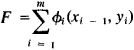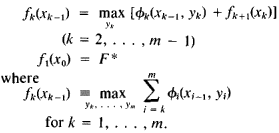# Dynamic Programming

Also found in: Acronyms, Wikipedia.

## dynamic programming

[dī¦nam·ik ′prō·grə·miŋ]
(mathematics)
A mathematical technique, more sophisticated than linear programming, for solving a multidimensional optimization problem, which transforms the problem into a sequence of single-stage problems having only one variable each.
McGraw-Hill Dictionary of Scientific & Technical Terms, 6E, Copyright © 2003 by The McGraw-Hill Companies, Inc.
The following article is from The Great Soviet Encyclopedia (1979). It might be outdated or ideologically biased.

## Dynamic Programming

a branch of mathematics devoted to the theory and methods of solving multistage optional control problems.

Dynamic programming attempts to find for a control process, that control from among all possible controls which provides the extreme (largest or smallest) value of a criterion function representing a certain numerical characteristic of the process. The term “multistage” refers either to the multistage structure of a process or to the breakdown of a control into a number of sequential stages (steps) corresponding, as a rule, to various moments in time. Thus, in so-called “dynamic programming,” the word “programming” is understood to mean “decision-making,” or “planning,” and the word “dynamic” indicates the essential role of time and the sequential performance of operations in the processes and methods under investigation.

The methods of dynamic programming are an integral part of the methods used in studying operations (operations re-search) and are used both in problems of optimal planning and in solving various engineering problems (for example, in determining optimal dimensions of multistage rockets, in routing roads optimally, and so on).

Suppose, for example, that the control process of a certain system consists of m steps (stages) and that at step i the control yi transforms the system from state xi - 1 to a new state xi, which depends onxi 1 and yi.

xi = xi(yi, xi - 1)

Thus, the control sequence y1, y2, … , ym transforms the system from an initial state x0 into a final state xm. It is necessary to select x0 and y1 … , ym such that the functionattains its maximum value F*. The basic method of dynamic programming is to reduce this general problem to a sequence of simpler extremal problems. By using the so-called principle of optimality formulated by the American mathematician R. Bellman, it is easy to obtain the basic recurrence equation:Thus, the method of dynamic programming makes it necessary to solve this recurrence relation. In the solution process, the sequence of stages is gone through twice: the first time from the end to the beginning (optimal values are found for F* and x0*) and the second time, from the beginning to the end (the optional control sequence y1*, … , ym* is found).

The methods of dynamic programming are used not only in discrete but also in continuous control processes, for example, in processes where decisions must be made at every moment of a certain interval of time. Thus dynamic programming has offered a new approach to problems in the calculus of variations.

Although the method of dynamic programming allows a radical initial simplification of many important problems, its direct application still entails cumbersome computations. Approximate methods of dynamic programming are being worked out to overcome these difficulties.

### REFERENCES

Bellman, R. Dinamicheskoe programmirovanie. Moscow, 1960. (Translated from English.)
Hadley, G. Nelineinoe i dinamicheskoe programmirovanie. Moscow, 1967. (Translated from English.)

V. G. KARMANOV